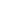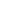# Numpy Square, Explained

In this tutorial, I’ll show you how to use the Numpy square function, which is sometimes called np.square.

The Numpy square function is fairly straight forward, but in the tutorial, we’ll explain everything step by step.

You’ll learn about how the syntax works, and we’ll also show you some clear examples.

You can click on any of the links above, and it will take you to the appropriate section.

Having said that, to get a full understanding of the function, you should probably read the whole thing.

## A quick introduction Numpy Square

Let’s start off with a quick introduction to the function.

As you probably know, the Numpy Square function is a function in the Numpy package in Python.

All of the functions in Numpy perform operations or computations on numeric data. That’s essentially what np.square does too.

The Numpy square function calculates the square of a numeric input.

So for any value x that we use as an input to the function, Numpy Square will calculate.In some ways, it’s similar to some other mathematical functions in Numpy like Numpy power, which computes exponents for input values, or Numpy log, which computes natural logarithms. Numpy square is similar to these other functions in the sense that they share a similar syntactical structure.

Having said that, let’s take a look at the syntax.

## The syntax of np.square

Here, I’ll explain the syntax of np.square. But before I do that, I need to make one quick note about importing Numpy.

#### A quick note about importing Numpy

Remember: whenever we use a Python module, we need to import that module first.

How we import a module will change how we write the syntax.

Having said that, in all of the following examples and explanations, we’ll assume that you’ve imported Numpy with the alias ‘`np`‘.

To do this, you can run the following code:

```import numpy as np
```

This is the common convention for importing and using Numpy, so we’ll be sticking with that here.

### Numpy square syntax

Ok.

As I mentioned previously, the syntax for the square function is very, very simple.

Typically, we’ll call the function as `np.square()`.Inside of the parenthesis, there is a parameter `x` that allows us to specify an argument to the function (i.e., an input on which Numpy will compute the square).

Let’s take a quick look.

###### `x` (required)

The `x` parameter enables you to specify the input to the function (i.e., the argument).

The input can be a single number, a Numpy array, or an “array-like” object. Array-like objects are things like Python lists or tuples.

###### Other parameters

Technically, there are other parameters for the square function, such as `out` and `where`.

These are used somewhat rarely, so we will not cover them in this tutorial.

## Examples of how to use Numpy square

Now that you’ve learned about the syntax, let’s take a look at some examples of Numpy square.

Examples:

#### Run this code first

Before you run any of these examples, you’ll need to import Numpy.

As explained in the syntax section of this tutorial, how we import Numpy will impact the exact syntax.

The common convention among Python data scientists is to import Numpy with the alias ‘`np`‘, and we’ll be using that convention here in these examples.

So before you run these examples, make sure that you import Numpy with the following code:

```import numpy as np
```

Ok. Now that you’ve done that, let’s get started.

### Example 1: Calculate the square of a single value

Where, we’ll calculate the square of the value 5.

```np.square(5)
```

OUT

```25
```
###### Explanation:

This is very simple.When we use Numpy square and provide a single number as the argument to the function, np.square simply computes the square of that value.

So when we use the value 5 as the input, np.square computes, which equals 25.

### EXAMPLE 2: Calculate squares of values in a 1D array

Next, we’ll compute the square of the values in a 1-dimensional Numpy array.

To do this, we’ll first create a simple Numpy array, and then we’ll use that as an input the Numpy square.

###### Create numpy array

Here, we’ll create a very simple Numpy array using the Numpy array function.

```array_1d = np.array([0,1,2,3,4])
```
###### Run np.square

Now that we have an array, we can run Numpy square.

```np.square(array_1d)
```

OUT:

```array([ 0,  1,  4,  9, 16])
```
###### Explanation

Again, this is fairly straight forward.

When we provide a 1-dimensional array to Numpy square, np.square computes the square of every element.### EXAMPLE 3: Compute the squares of values in a 2D array

Finally, let’s use Numpy square on a 2-dimensional array.

This really works the same as computing the values in a 1D array. The only major difference is the structure of the input.

###### Create 2D array

First, we’ll create a 2-dimensional Numpy array.

We’ll create an array with the values from 1 to 9, arranged in a 3-by-3 shape.

To do this, we’ll use the Numpy arange function in combination with the Numpy reshape method.

```array_2d = np.arange(start = 1, stop = 10).reshape((3,3))
```

Now that we have our 2D array, let’s use Numpy square.

###### Run the square function

Here, we’re simply going to provide the name of the array, `array_2d`, as the input to `np.square()`.

```np.square(array_2d)
```

OUT:

```array([[ 1,  4,  9],
[16, 25, 36],
[49, 64, 81]])
```
###### Explanation

Again, this is fairly straight forward.

When we provide a Numpy array as an input to np.square, thefunction will complete the square of every value of the array, element wise.Do you have questions about Numpy square?

The examples here about Numpy square are pretty simple and easy to understand.

But other parts of Numpy can be a lot more complicated.

If you’re serious about learning Numpy (and serious about data science in Python), you should consider joining our premium course called Numpy Mastery.

Numpy Mastery will teach you everything you need to know about Numpy, including:

• How to create Numpy arrays
• How to use the Numpy random functions
• What the “Numpy random seed” function does
• How to reshape, split, and combine your Numpy arrays
• How to perform mathematical operations on Numpy arrays
• and more …

Moreover, it will help you completely master the syntax within a few weeks. You’ll discover how to become “fluent” in writing Numpy code. If you have trouble remembering Numpy syntax, this is the course you’ve been looking for.

Find out more here:

Joshua Ebner

Joshua Ebner is the founder, CEO, and Chief Data Scientist of Sharp Sight. Prior to founding the company, Josh worked as a Data Scientist at Apple. He has a degree in Physics from Cornell University.

### 2 thoughts on “Numpy Square, Explained”

1. The tutorial is quire extensive and easy to understand